• Shuffle
Toggle On
Toggle Off
• Alphabetize
Toggle On
Toggle Off
• Front First
Toggle On
Toggle Off
• Both Sides
Toggle On
Toggle Off
Toggle On
Toggle Off
Front

### How to study your flashcards.

Right/Left arrow keys: Navigate between flashcards.right arrow keyleft arrow key

Up/Down arrow keys: Flip the card between the front and back.down keyup key

H key: Show hint (3rd side).h key

A key: Read text to speech.a keyPlay buttonPlay buttonProgress

1/48

Click to flip

### 48 Cards in this Set

• Front
• Back
 What are the engineering notations nomenclatures? Tera, Giga, Mega, Kilo, E12 E9 E6 E3Pico, Nano, Micro, Mili, E-12 E-9 E-6 E-3 What is the equation to convert voltage gain (Av) into dB (Av') Av'=Av * 20LOG LM741 symbol and Pinout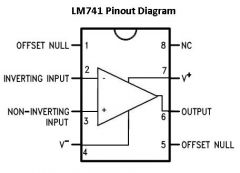What are the characteristics of an ideal Op-Amp Infinite gainInfinite input impedance0 output impedance what is a single ended mode Op-amp?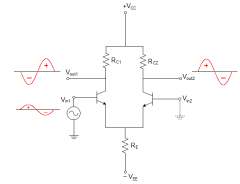Only on of the inputs is grounded and the signal is applied to the other input. what is a differential mode Op-amp?what is a common mode Op-amp?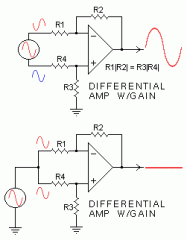(dif)-2 out of phase signals are applied to the inputs.(common)- in phase signals are applied to both inputs and a cancel/very small signal is the output. How do you calculate CMRR (common mode rejection ratio)? CMRR= Av (d)/ Av(cm) CMRR'= 20Log( Av(d)/ Av(cm)) Input bias and offest current... Input bias = I1+I2/ 2 "average current"Input offeset current= |I1- I2| What are inverting and non-inverting amplifiers?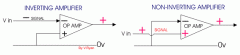Non-invert: Av= (Rf/Ri) + 1invert: Av= -Rf/Ri How do you calculate output voltage if you know the input voltage and the gain? Vout = Av * Vin What are the input and output impedance's of an inverting (I) and non-inverting (NI) amplifiers? ( I ) -input : Zin = Ri -output: Z out = Z out/ (1 +Aol*B)( NI ) -input: (1+ Aol*b) Z in -output: Zout/ (1+Aol*b) How does bandwidth relate to an Op-amp? BW= F(cu) - F(cl) Note: since op-amps do not have a lower critical frequency(F(cl)), the banwidth can simply be written as :BW= F(cu) "upper critical freq." Define: Unity gain frequency, Critical frequency, and mid-range gain. Unity Gain Freq.: when the gain is effectively 0dB, or Av = 1, at a given frequency.Critical frequency: when the gain is effectively -3dB less than the mid-range gain.Mid-range Gain: Could also be defined as open loop gain (Aol). What is Gain-Bandwidth Product (GBP)? GBP is the same thing as the UGB "unity gain bandwidth.if: Acl*Fcl = Aol * Folthen: Acl*Fcl = Unity gain BW/ GBP Explain the difference between Positive (+) and Negative(-) feedback. Positive FB: required to make an op-amp circuit oscillate. Positive feedback is effectively 0-360 degrees out of phase.... basically the output is in phase with the input.Negative FB: when applied to the (+)/(-) inputs, they are almost identical. Negative feedback is an excellent way to control gain. The output is typically going to be out of phase with the input, to what degree, it varies with different configurations. what is the definition of a comparator?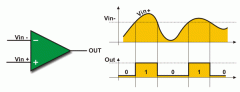A comparator compares two voltages and saturates the output depending on the comparison. What is noise and how does it effect a comparator? How do you get rid of it? Nois on the input of a comparator is a superimposed of "riding" signal on the original input. This can cause severe output fluctuation and the best way to eliminate it is to add Hysteresis.Hysteresis is just a technique that incorporates positive feedback. What is a "Window Comparator"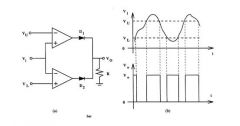A Window Comparator detects when and input voltage is withing the two threshold limits it sets for itself, Vu & Vl. When Vin goes above or below Vu or Vl, it goes into saturation. describe: Summing amplifiers Summing amps add together their collective inputs. (same R) Vout= -(Vin1+ Vin2 +Vin3....etc)(if Rf is > Rin) Vout= -(Rf/R)(Vin1+Vin2+Vin3... etc) describe: Averaging Amplifiers An Averaging Amplifier produces a mathematical average of the input voltages by setting the ration of Rf/R equal to the # of inputs.Note: the equations are the same as a summing amp. describe: Scaling Adder Vout= - ((Rf/R1)Vin1 + (Rf/R2)Vin2 + (Rf/R3)Vin3...etc)Note: the scaling adder is used when the R, and the Vin, of the inputs are all different values. Filters: Low pass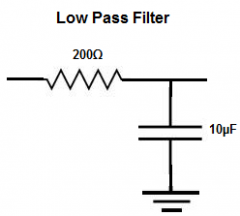The passband of a lowpass filter allows all freq. lower than the critical frequency (Fc)Fc= BW= 70.7% Fin= Av'-3dBFc= 1/(2pi R*C) Fliters: High Pass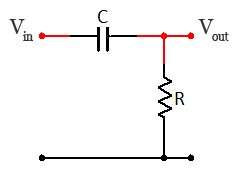Attenuates frequencies below Fc and allows all frequencies above Fc to pass.Fc= 1/(2pi R*C) Filters: Band Pass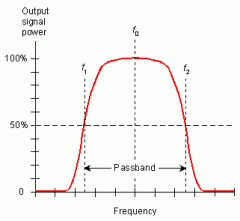Allows only a certain band of frequencies to pass while attenuating frequencies below Fc1 and above Fc2. BW= Fc2 - Fc1F0=sqrt Fc1*Fc2Q= F0/BW =approx... 1/DF "dampening factor" Filters: Band Stop "notch"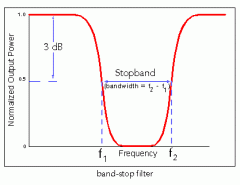Allows most, if not all freq. while attenuating a certain band or even just a specific freq., depending on the quality. What is the "Q" of a filter? "Q" or Quality factor of a filter is define as the ration of the center freq., to the bandwidth.Q= F0/BW What is the Dampening Factor, and how is it calculculated? Dampening factor (DF): Determines the filter respose, ( whether it is a chebyshev, bessel or butterworth response)DF = 2-(R1/R2) RC Poles and filter order...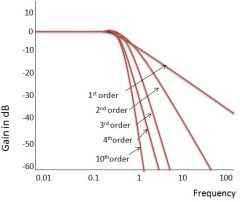refer to butterworth response requirements handout how does a cascaded high/low pass filter make a bandpass filter?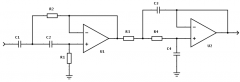-Fc1= 1/(2pi * sqrt Ra1Rb1Ca1Cb1)-Fc2= 1/(2pi * sqrt Ra2Rb2Ca2Cb2)-F0= sqrt Fc1*Fc2 active low pass filter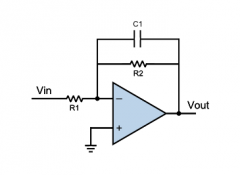active high pass filter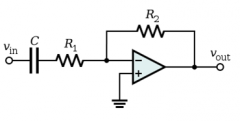active band pass filter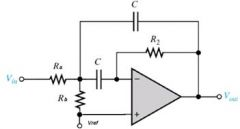What is the difference between an amplifier and an oscillator? Amplifiers: use gain from the feedback network, open loop or closed loop, to produce a greater signal. uses negative feedback.Oscillators: Turn DC input into a waveform utilizing positive feedback. What are the conditions for Oscillation? 1. Phase shift must be 02. Voltage gain must equal 13. in order for oscillation to begin, the closed loop voltage gain must be greater than 1. After this, it must revert back to a voltage gain of 1. What are the formulas and operation of a Wien-Bridge oscillator. Gain (AV)=Vout/Vin= 1/3Fr= Resonant Freq. = 1/(2pi R*C)note: it generates a large range of stable frequencies. what is a relaxation oscillator? Uses and RC Timing circuit and a device that changes states to make a periodic wave (non-sinusoidal).... basically an oscillator that makes square waves. What is a voltage controlled oscillator? A relaxation oscillator whose frequency is controlled by a variable DC control voltage. note: remember project 2 What is the difference between a-stable and monostable devices? A-stable: a circuit that alternates between two unstable states.Monostable: has only one stable state. what is an a-stable multivibrator?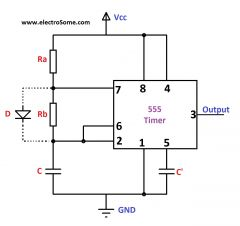Duty cycle: ((R1+R2)/(R1+2R2))*100note: placing a diode over R2, in parallel, allows the duty cycle to be lower than 50% what is a monostable multivibrator?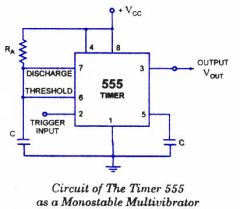note: a.k.a. "one-shot" Series Regulator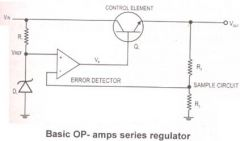Shunt Regulator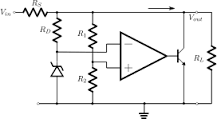Switching regulators (stepup, stepdown, inverting)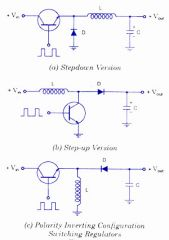Vout= (t on/ T)Vin what are the basic external components of an IA, "Instrumental Amplifier?" Resistors are the main external components that are used for feedback networks and to set gain. What is an Operational Trans-conductance amplifier used for? OTA 1. Amplitude modulator2. Schmitt Trigger How do you calculate gain in dB for multiple stage filters. Av'= 20log(Vout/Vin)Av' tot= Av'1+ Av'2+ Av'3... etcnote: when adding the gain is in dB form, it is the equivalent of multiplying the original gain ration.Av' tot= Av'1 + Av'2 + Av'3 = Av1 * Av2 * Av3 How do you calculate Xc, capacitive reactance for any given freq. if you are give Fc, and R in a filter network? Fc= 1/(2pi R*C)C = 1/(2pi Fc*R)R = 1/(2pi Fc*C) = Xc How do you calculate the phase shift through a at any given freq. if you are give Fc? Φ = -tan^-1(f/FC)Φ = phase shift...